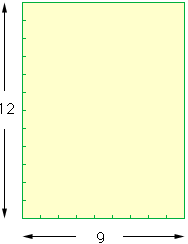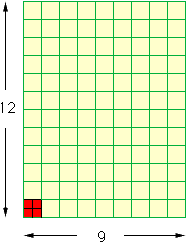Hello. My name is Carolyn Clarkston. I am a parent of a middle school (8th grade) child who is preparing for the state LEAP test next year. There is a math problem that I'm having trouble helping him with. Please give me not only the answer but the explanation behind it. Thank you. The problem: A square tile measures 6 inches by 6 inches. What is the least number of tiles needed to cover a rectangular floor area of 9 feet by 12 feet? Hi Carolyn, I would draw a picture - and notice the units. If you draw a picture, with everything in inches you will have something which is 108 inches by 144 inches. Alternatively, you can draw it 9 by 12 and notice that the tiles are .5 by .5 in feet.Now, consider going across the bottom - 9 feet or 108 inches. This will take 18 of the tiles. Cosider going up the side - 12 ft or 144 inches. This will take 24 tiles. For the entire rectangle, you now need 18x24 tiles. IF you drew the floor on grid paper, with one foot for each two square along the sides and bottom, you will actually SEE a square for each tile (four tiles per square foot). This reinforces the imagery. There are 9x12=108 square feet of floor so seen this way you need 4x108 tiles.You COULD do it by pure arithmatic: area = 9x12 sq ft. Area of tile = .5x.5 sq ft Divide total area by area of tile to get number of tiles. The answers will be the same, but I think it helps to 'see the picture' then perhaps compare the two methods for understanding how algebra represents the picture! Ideally, someone can do it both ways and understand the connections. Walter Whiteley Go to Math Central